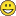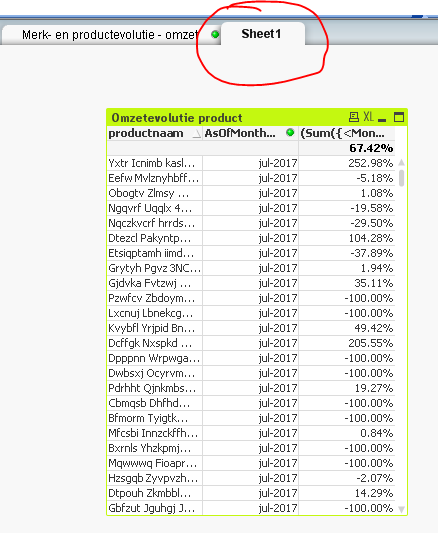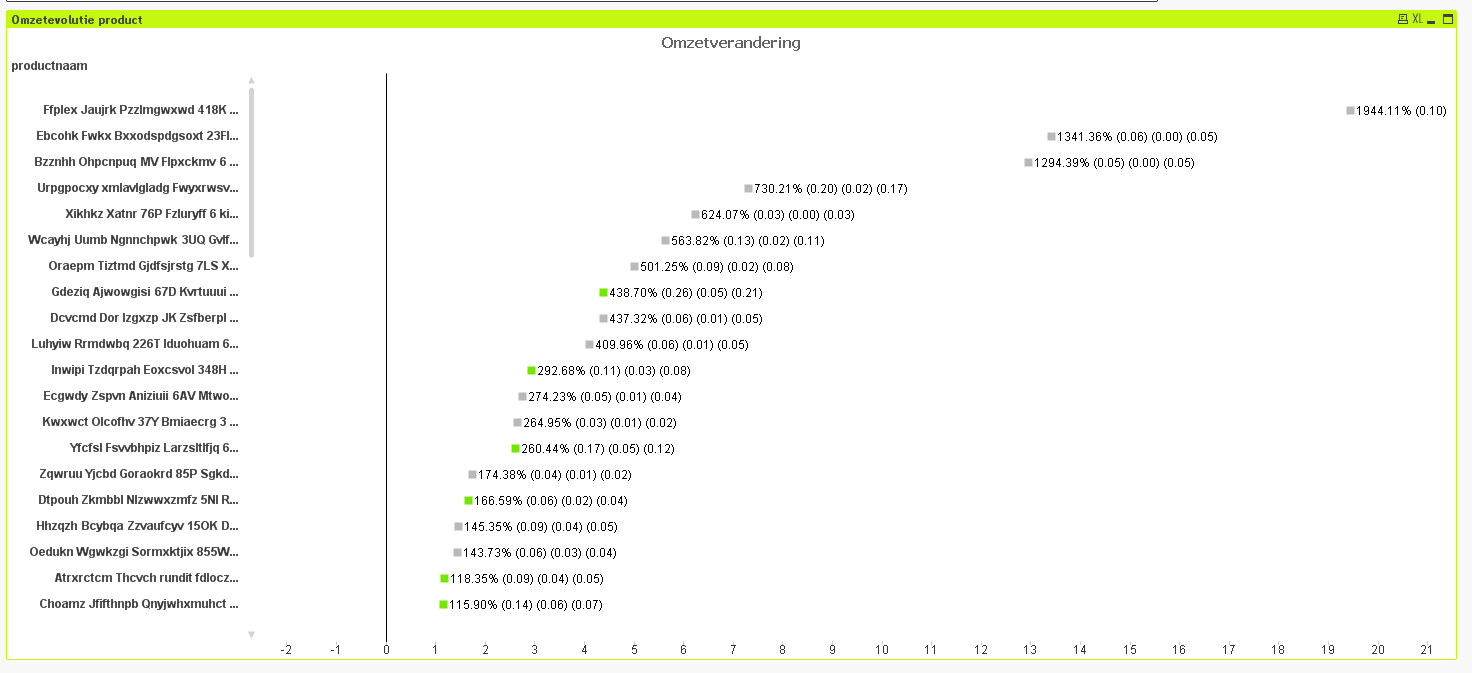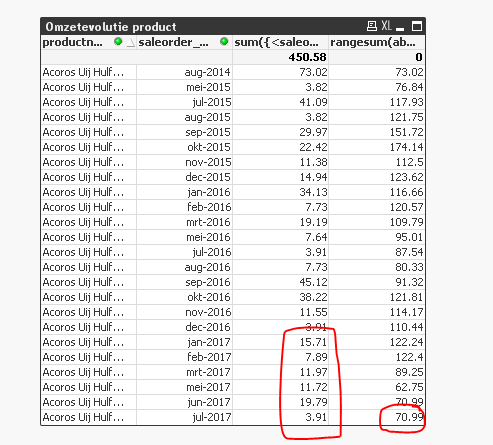# QlikView App Dev

Discussion Board for collaboration related to QlikView App Development.

Announcements
cancel
Showing results for
Did you mean:Contributor III

## Rangesum expressions in combination with If (RowNo ...) not working

Hi guysSo I have a question that I know will probably be not that easy. I will add a data file for sure and I will give all information that I can possibly give.

So on the main sheet you will find some graphs/lists/boxes. Let me quickly address them all since they are in DutchThe first one are the months that I use to calculate revenue. They should be similar to English so you should be able to understand those.

Then there is a current selections box which is basic so no problem there.

Sale_order website is scrambled so just ignore.

In te vullen variabelen VOOR MERK: this is a box I use to make variables and give them values. All 6 are used in the first graph (Omzetevolutie merk).

First one: How much the brand must increase in order to be showed in the graph over a certain period.

Second: Same as first but not the limit so if a brand has risen more than this value, it will not be showed.

Third: Minimal revenue for the FIRST period

Fourth: Minimal revenue for the SECOND period

Fifth: The amount of months each period has. 6 means: we want to compare a total of 6 months to another total of 6 months.

Sixth: The amount of time between the 2 periods.

For example: fifth = 6 and sixth = 0 means that we want to compare 6 months to the previous 6 months.

This only works if the selected months are done right. After a lot of experimenting, I came up with a formula that made this work in every case, no matter how long the periods/time between periodes (but ofcourse within the possible time of the file so not more than 40 something months). So for 6 and 0 (fifht/sixth variable) I need to select 12 months.

Productnaam list is the list of products with their name. They are scrambled however. There is also 1 brand in the file: Mbrrbb (scrambled) so this is the only brand to work with.

The first graph shows evolution of this brand in revenue over a certain period. For the 6 and 0 this means that the last 6 selected months, have a revenue of 25.88 thousand and the 6 months before had 15.46 thousand. The difference is 10.42 thousand and this is an increase of 67.42% (these values are all shown in the graph next to the datapoint). Note that changing the variables could result in omitting this brand so keep the minimum revenues as low as possible (1000 will do) and keep the min and max increase high (so -100% and like 1000% for the max change so that this does not interrupt anything).

The second one shows evolution of all products within this brand. But, and this is the problem: it does not show all products of this brand. Only the ones that have 12 data points (so 1 value for every selected month) are calculated. The others (with 11 or less values) are not calculated because of how the expression is created (with RowNo() >= ....).

My question now is: how can I have all products listed in there, not matter how much data points they have? And they have to be seperated in the right way ofcourse. If the product has 1 value in the first 6 months and 2 values in the next 6 months, it should calculate the difference between those 2 periods correctly without mixing the values up in the different periods.

This is a hard question I guess so if u need more material or some example of what the values should be or the output, just ask and I'll see what I can doKind regards and thanks in advance!!!

1 Solution

Accepted SolutionsMVP

Check the attached14 RepliesMVP

Def. not one of the easier questions, but I am up for the challenge. So, I am going to convert your combo chart into a straight table as I can see the numbers more clearly in it compared to a chart. For fixing this.... I am seeing 8 productnaam, but it seems that you are hoping to see more productnaam? Is that right? How many more more do you expect to see? All possible values that show in productnaam list box?MVP

Not 100% sure, but does this give you what you are looking to get?

if(saleorder_monthyear = Max(TOTAL saleorder_monthyear),

dual(round(100*(rangesum(above(sum({<saleorder_monthyear>}saleorder_netamount),0,Aantalmaandenperiode))-rangesum(above(sum({<saleorder_monthyear>}saleorder_netamount),AantalMaanden+Aantalmaandenperiode,Aantalmaandenperiode)))/rangesum(above(sum({<saleorder_monthyear>}saleorder_netamount),AantalMaanden+Aantalmaandenperiode,Aantalmaandenperiode)),0.01)

&'% (' & round(rangesum(above(sum({<saleorder_monthyear>}saleorder_netamount),0,Aantalmaandenperiode))/1000,0.01) & ') ('

& round(rangesum(above(sum({<saleorder_monthyear>}saleorder_netamount),AantalMaanden+Aantalmaandenperiode,Aantalmaandenperiode))/1000,0.01) & ') ('

& round(((rangesum(above(sum({<saleorder_monthyear>}saleorder_netamount),0,Aantalmaandenperiode))-rangesum(above(sum({<saleorder_monthyear>}saleorder_netamount),AantalMaanden+Aantalmaandenperiode,Aantalmaandenperiode)))/1000),0.01) & ')',

(rangesum(above(sum({<saleorder_monthyear>}saleorder_netamount),0,Aantalmaandenperiode))-rangesum(above(sum({<saleorder_monthyear>}saleorder_netamount),AantalMaanden+Aantalmaandenperiode,Aantalmaandenperiode)))/rangesum(above(sum({<saleorder_monthyear>}saleorder_netamount),AantalMaanden+Aantalmaandenperiode,Aantalmaandenperiode))))Contributor III
Author

Hi youSo I want to be able to see all of them by scrolling down, I usually change the chart so that I can see 5 items and if there are more than 5, I can scroll down to see other items. The screenshot looks promising but I can only check it next friday.

Thanks though, I assume this is the solution and it's quite great that you figured it out alreadyHow does it work though? I see you changed the first line but why is it able to calculate it now? And does it work for a few other combinations of periods/time between periods?

The color of the dots I will write myself because it's quite easy once you have the correct expression haha.Contributor III
Author

Hey again

Well, I should have noticed ofcourse, but this does not work (unfortunately). You can easely see this too since all the products in the screenshot have extremly low values for sales. It seemed as the perfect solution but wellI wil help you though. I will upload a new QV file with an extra table. This table shows sales for the last 12 months so you can manually verify the % in excel. If you download this table in excel and sum the first 6 months and the second 6, you can calculate the percentual difference. Also, just clicking on one item gives the table for the 1 item and you can immediatly see that the %'s are not correct.

I also understood what you did with the set expression now. Perhaps that the arguments of the rangesum should be changed too? I ask almost in every rangesum to take 12 months (2 times the 6 month period + the time in between which is 0 in this example so 2*6 + 0 = 12). Shoudln't this be changed to something like Max(total saleorder_monthyear then too?)

Thanks for the effort and hopefully you haven't given up on meKind regards

Ps: if you would try this formula, with all the current if's that correspond with the variables in the table, would you still get the (correct) 65% for the mark (haven't tested that). This could also be an idea to check whether or not the expression worksMVP

Lets pick a productnaam, can you tell me what the expected output needs to be for this guy?Contributor III
Author

So the calculation should make the sum of the first 6 months and calculate the percentual difference between those 6 months and the next 6 months. For this product, this means that the first 6 months are:

Juli 2017

June 2017

May 2017

April 2017

March 2017

February 2017

The total sum for these months is: 3.91 + 19.79 + 11.72 + 11.97 + 0 (April 2017 is not in the screenshot) + 7.89 = 55.28

The 6 months before this period gives a total of 122.24 if I calculated correctly.µ

So the output in graph has to be:

First the percentage that gives the difference. Calculation is as follows:

(55.28 - 122.24)/122.24 = -0.54777 ... or -54.78%.

The number right next to this percentage, should be the total sum of the last 6 months divided by 1000 or for this example: 55.28/1000 = 0.05528 rounded = 0.06

The number next to that should be the total sum of the first 6 months so: 122.24/1000 = 0.12224 = 0.12

The last number gives the difference in absolute values: 0.06-0.12 = -0.06

Does this clarify what I want to achieve with every product?MVP

I think the problem is missing values which is causing RangeSum() to not accumulate correctly... Take a look hereSince there is no April, RangeSum(...., 0, 6) is considering Jan 2017 into the calculation.... RangeSum have no idea that April is a 0 and just look at the last 6 values and sum them up....

Now there are 2 ways you can address the issue

1) Populate the missing values

Generating Missing Data In QlikView

2) Use AsOfTable

Although, I like the AsOfTable approach, it might become challenging in your case because you have a dynamic way to determine how many periods back the user can go based on user input....Don't get me wrong, it is still possible to do this way, but might have to be played and tested with....

Both methods will have there challenge, but you will have to decide which way you would like to go...Contributor III
Author

No offense, but this was exactly what the issue was, and I described this as being the issue!I copy from my initial post:

Copied text:

The second one shows evolution of all products within this brand. But, and this is the problem: it does not show all products of this brand. Only the ones that have 12 data points (so 1 value for every selected month) are calculated. The others (with 11 or less values) are not calculated because of how the expression is created (with RowNo() >= ....).

This was not in the original post: I should have mentioned that it was also a problem for the rangesum indeed!

Copied text:

My question now is: how can I have all products listed in there, not matter how much data points they have? And they have to be separated in the right way of course. If the product has 1 value in the first 6 months and 2 values in the next 6 months, it should calculate the difference between those 2 periods correctly without mixing the values up in the different periods.

End of copied text.

I think that the second method would be possible but I do not know how to implement it. I actually came across this post and just skipped it because I am not experienced enough to understand this (since I did not write the script and only do the QV calculations/graphs/...). So would you be able to solve it or am I on my own?MVP

No offence taken my friend...

With regards to helping... I do want to help... but there are few questions and concerns

1) How many possible values can the user input for rangeSum input? We are using 6 right now... how high and low can it go? Will it go in certain increments?

2) I don't have the source files... I have a way around that... but can (and probably will) take some extra time for me to code it....